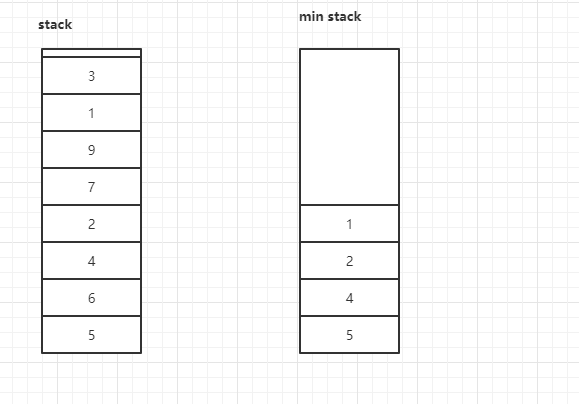• 😁 第一次练习 2020年3月17日 五毒神掌
• 💩 第二次练习 2020年5月12日 过了一个月，思路还是记得，以前是用 JavaScript 做的 现在用 Java 实现，不过需要注意Java 有 equals 问题。 两次入栈法也用 Java 实现了一次

### # 利用两个栈``````class MinStack {
private Stack<Integer> stack;
private Stack<Integer> minStack;
/** initialize your data structure here. */
public MinStack() {
stack = new Stack<>();
minStack = new Stack<>();
}

public void push(int x) {
stack.push(x);
if (minStack.isEmpty() || minStack.peek() >= x) {
minStack.push(x);
}
}

public void pop() {
if (stack.isEmpty()) {
return;
}

if (stack.pop().equals(minStack.peek())) {
minStack.pop();
}
}

public int top() {
return stack.peek();
}

public int getMin() {
return minStack.peek();
}
}
``````

### # 两次入栈法

``````class MinStack {
private Stack<Integer> stack;
private Integer min;
/** initialize your data structure here. */
public MinStack() {
stack = new Stack<>();
min = Integer.MAX_VALUE;
}

public void push(int x) {
if (x <= min) {
this.stack.push(min);
min = x;
}
this.stack.push(x);
}

public void pop() {
if (stack.isEmpty()) {
return;
}

if (stack.pop().equals(min)) {
min = stack.pop();
}
}

public int top() {
return stack.peek();
}

public int getMin() {
return this.min;
}
}
``````

### # 易错点

• 需要注意，在判断元素大小的时候，需要考虑到等于的情况。
• 注意条件判断问题。
• 使用一个栈的方法时需要注意，需要先处理最小值问题，在入栈本来的元素，顺序不可颠倒